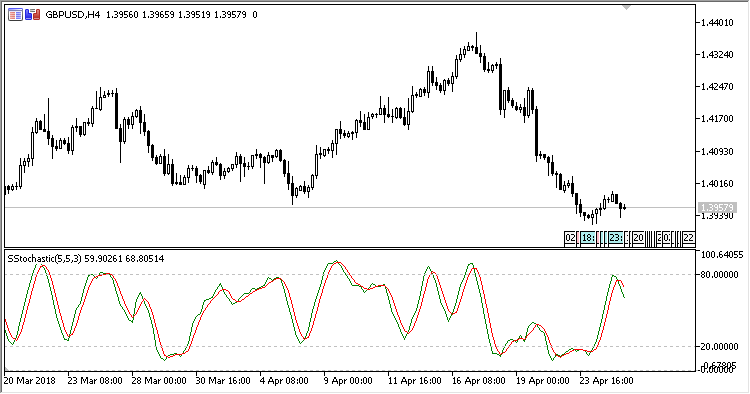# Stochastic_Slow – indicator for MetaTrader 5

• A+
category：MT5 INDICATORS

The Slow Stochastic oscillator shows in a separate window a stochastic calculated as follows:

`SSD[i] = SMA(FSK,PeriodD)`
`SSDS[i] = SMA(SSD,Slowing)`

where:

```FSK[i] = (min / max) * 100.0,
SSD[i] = SMA(FSK,PeriodD),
min = close[i] - low[BL],
max = high[BH] - low[BL],
BH is the highest price index within the range from PeriodK to i,
BL is the lowest price index within the range from PeriodK to i,
```

if max = 0,

`FSK[i] = 50.0`

The indicator has five input parameters:

• Period K - the period of calculating line K;
• Period D - the period of calculating line D;
• Slowing - the slowing period;
• Overbought - overbought level;
• Oversold - oversold level.